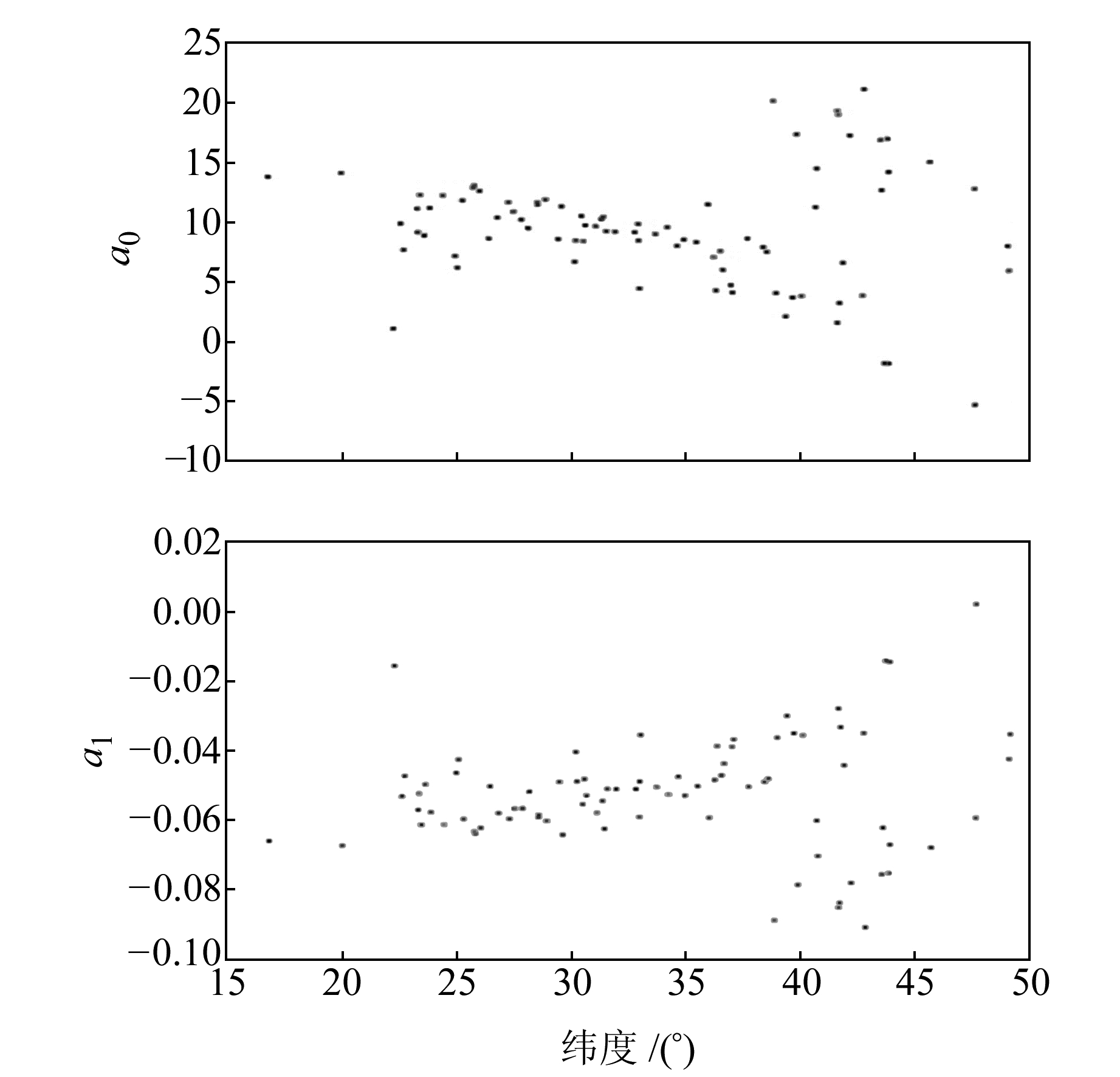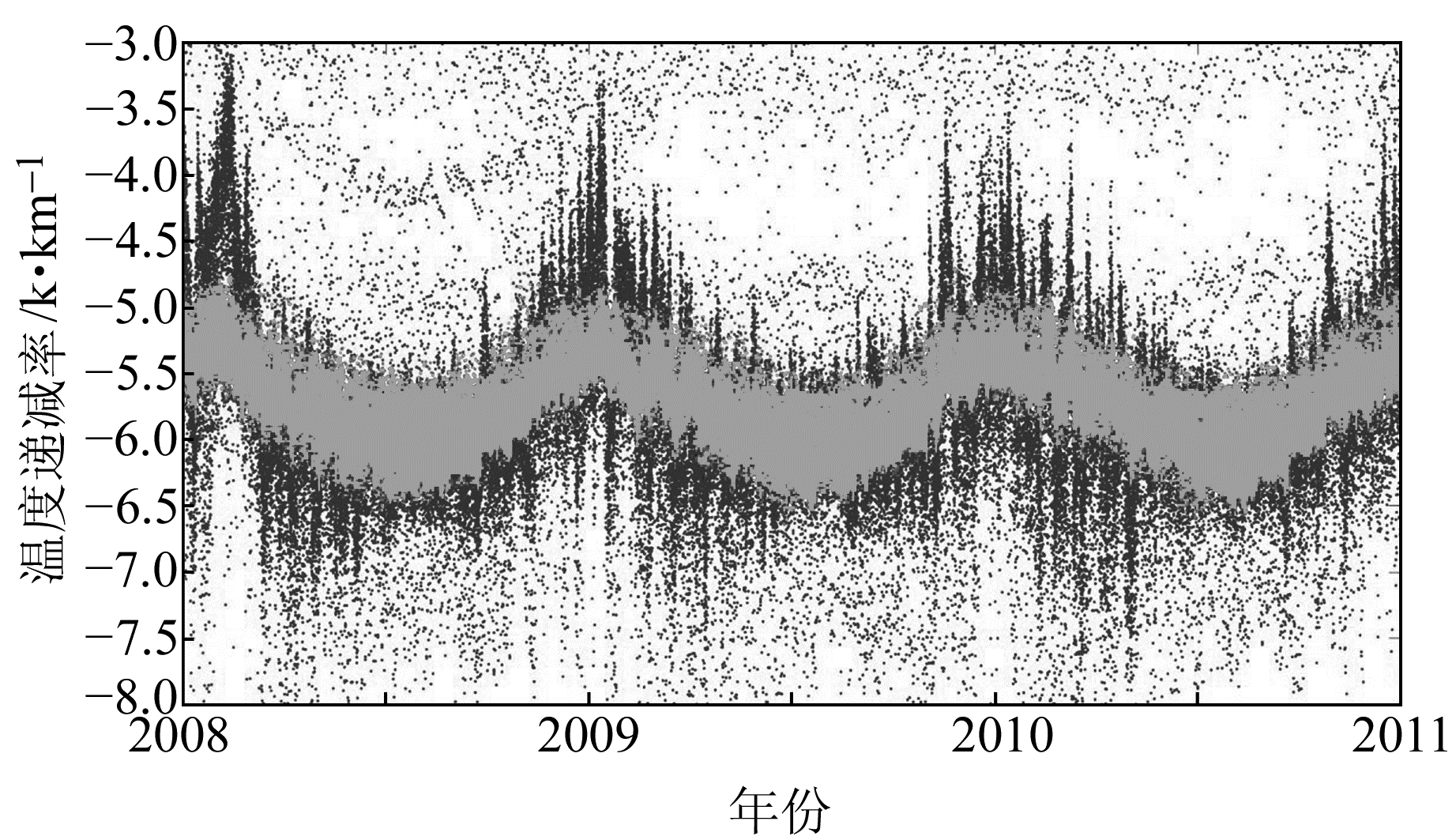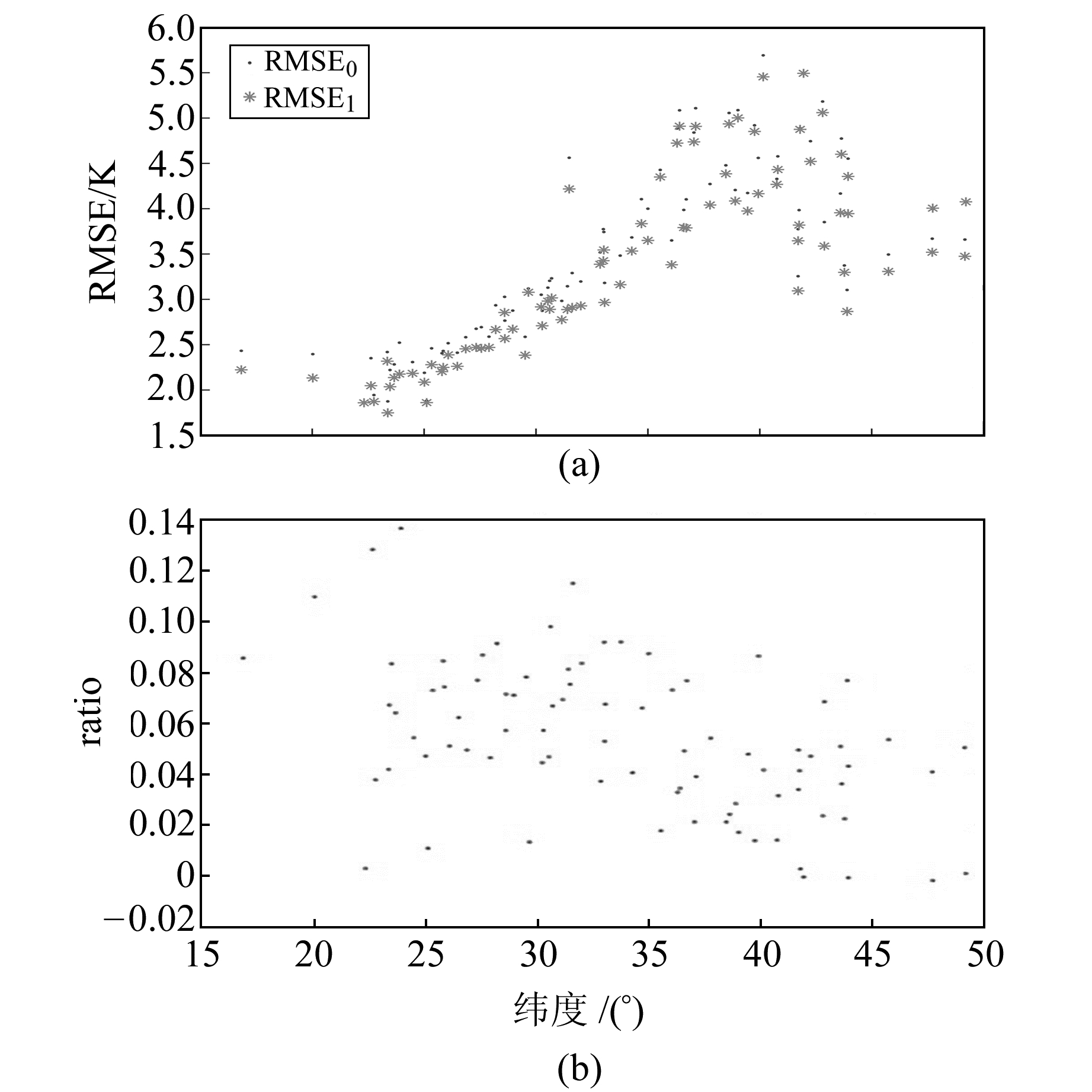﻿ 温度递减率对加权平均温度的影响文章快速检索 高级检索
 大地测量与地球动力学2019, Vol. 39Issue (6): 639-642  DOI: 10.14075/j.jgg.2019.06.017### 引用本文LIU Jinghong, YAO Yibin, SANG Jizhang. Temperature Lapse Rate and Its Influence on Weighted Mean Temperature[J]. Journal of Geodesy and Geodynamics, 2019, 39(6): 639-642.### Foundation support

National Natural Science Foundation of China, No. 41574028.

### Corresponding author

YAO Yibin, PhD, professor, PhD supervisor, majors in GNSS meteorology, E-mail:ybyao@whu.edu.cn.

### 第一作者简介

LIU Jinghong, PhD candidate, majors in GNSS meteorology and atmospheric re-entry, E-mail:liu-jh@whu.edu.cn.

### 文章历史

1. 武汉大学测绘学院，武汉市珞喻路129号，430079

1 温度递减率模型的建立

 $T = {T_s} + \delta h$ (1)

 $\delta = \frac{{\sum\limits_{i = 1}^n {\left( {{T_i} - \bar T} \right)} \left( {{h_i} - \bar h} \right)}}{{\sum\limits_{i = 1}^n {{{\left( {{h_i} - \bar h} \right)}^2}} }}$ (2)
1.1 探空站温度递减率的分析表 1 4个探空站的地理信息 Tab. 1 Locations of four radiosonde stations

 $\delta = {a_0} + {a_1}{T_s}$ (3)表 2 4个探空站的假设检验信息 Tab. 2 Hypothesis testing information

4个探空站的布尔变量都为0，表明残差的均值为0的假设是合理的，95%的置信区间完全包括0均值，且精度很高。从表 3也可以发现，随着纬度的升高，精度有降低的趋势; sig值均为1，远超过0.5。以上检验表明，式(3)的拟合残差属于正态分布，具有合理性。线性拟合结果见表 3表 3 4个探空站的线性拟合结果 Tab. 3 The results of linear fitting图 1 线性拟合系数a0和a1随纬度的变化 Fig. 1 Variation of a0 and a1 with latitudes
1.2 区域温度递减率模型的建立

 $\delta = {b_0} + {b_1}\varphi + \left( {{b_2} + {b_3}\varphi } \right){T_s}$ (4)图 2 温度递减率的拟合值和真值随时间的变化情况 Fig. 2 The fitting results varying with time图 3 RMSE和ratio随纬度的分布 Fig. 3 The distribution of RMSE and ratio with latitudes

2 温度递减率在加权平均温度中的应用

 $\begin{array}{l} {T_m} = aT_s^2 + a\delta \left( {{h_s} + {h^\prime }} \right){T_s} + \frac{{b\delta \left( {{h_s} + {h^\prime }} \right)}}{{{T_s}}} + \\ \;\;\frac{{b{{\left( {\delta \sqrt {h_s^2 + {h^{\prime 2}}} } \right)}^2}}}{{3T_s^2}} + \frac{{a{{\left( {\delta \sqrt {h_s^2 + {h^{\prime 2}}} } \right)}^2}}}{3} + b \end{array}$ (5)

 ${T_m} = {c_1}T_s^2 + {c_2}{T_s} + \frac{{{c_3}}}{{{T_s}}} + \frac{{{c_4}}}{{T_s^2}} + {c_0}$ (6)

 ${T_m} = {d_1}T_s^2 + {d_2}\delta {T_s} + \frac{{{d_3}\delta }}{{{T_s}}} + \frac{{{d_4}{\delta ^2}}}{{T_s^2}} + {d_5}{\delta ^2} + {d_0}$ (7)

 ${\rm{ratio}} = \frac{{{\rm{RMS}}{{\rm{E}}_0} - {\rm{RMS}}{{\rm{E}}_1}}}{{{\rm{RMS}}{{\rm{E}}_0}}}$ (8)

3 结语

  Angell J K, 周国华. 1960~1985年对流层和平流层低层全球温度的年、季变化[J]. 气象科技, 1988(1): 52-58 (Angell J K, Zhou Guohua. Annual and Seasonal Variations of Global Temperature in the Troposphere and Lower Stratosphere from 1960 to 1985[J]. Meteorological Science and Technology, 1988(1): 52-58) (0)  李启泰. 对福安地区温度垂直递减率的一些分析[J]. 农业气象, 1980, 1(4): 50-55 (Li Qitai. Some Analysis of Vertical Decline Rate of Temperature in Fu'an Area[J]. Chinese Journal of Agrometeorology, 1980, 1(4): 50-55) (0)  罗贵东, 夏利, 古书鸿. 四川省各温度范围内积温特征分析[J]. 贵州气象, 2016, 40(6): 42-47 (Luo Guidong, Xia li, Gu Shuhong. Analysis of the Characteristics of Accumulated Temperature in Each Degree Celsius in Sichuan[J]. Journal of Guizhou Meteorology, 2016, 40(6): 42-47 DOI:10.3969/j.issn.1003-6598.2016.06.008) (0)  郭劲秋.中低层大气温度和气溶胶廓线的拉曼激光雷达探测研究[D].南京: 南京信息工程大学, 2012 (Guo Jingqiu.The Detection of Temperature and Aerosol Profiles in Middle and Lower Atmosphere Using Raman Lidar[D]. Nanjing : Nanjing University of Information Science & Technology, 2012) http://cdmd.cnki.com.cn/Article/CDMD-10300-1012369307.htm (0)  姚宜斌, 刘劲宏, 张豹, 等. 地表温度与加权平均温度的非线性关系[J]. 武汉大学学报:信息科学版, 2015, 40(1): 112-116 (Yao Yibin, Liu Jinghong, Zhang Bao, et al. Nonlinear Relationship between the Surface Temperature and the Weighted Mean Temperature[J]. Geomatics and Information Science of Wuhan University, 2015, 40(1): 112-116) (0)  高攀, 徐晓华, 张小红, 等. 利用GPS掩星弯曲角确定对流层顶高度[J]. 武汉大学学报:信息科学版, 2012, 37(12): 1 417-1 420 (Gao Pan, Xu Xiaohua, Zhang Xiaohong, et al. Identification of Tropopause Height from GPS Radio Occultation Bending Angle Profile[J]. Geomatics and Information Science of Wuhan University, 2012, 37(12): 1 417-1 420) (0)  徐晓华, 高攀, 张小红. 利用COSMIC掩星弯曲角数据分析中国区域对流层顶结构变化[J]. 地球物理学报, 2013, 56(8): 2 531-2 541 (Xu Xiaohua, Gao Pan, Zhang Xiaohong. Structure and Variation of the Tropopause over China with COSMIC Radio Occultation Bending Angles[J]. Chinese Journal of Geophysics, 2013, 56(8): 2 531-2 541) (0)
Temperature Lapse Rate and Its Influence on Weighted Mean Temperature
LIU Jinghong1YAO Yibin1SANG Jizhang1
1. School of Geodesy and Geomatics, Wuhan University, 129 Luoyu Road, Wuhan 430079, China
Abstract: We establish a regional model of temperature lapse rate. After analyzing the statistic characteristics of the new model, we apply it to assess the effects of temperature lapse rate on the weighted mean temperature. The results show the high precision of temperature lapse rate is conducive to improving the distribution of the weighted mean temperature, which improves the accuracy of the PWV conversion of GPS in meteorology.
Key words: temperature lapse rate; surface temperature; weighted mean temperature